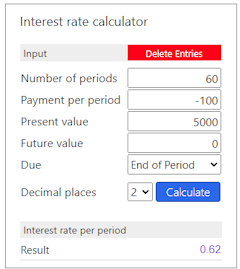# Rate Function

Rate returns a value specifying the interest rate per period for an annuity.

## Description of the function Rate

Returns a value specifying the interest rate per period for an annuity.

An annuity is a series of fixed cash payments made over a period of time. An annuity can be a loan (such as a home mortgage) or an investment (such as a monthly savings plan).

For all arguments, cash paid out (such as deposits to savings) is represented by negative numbers; cash received (such as dividend checks) is represented by positive numbers.

Rate is calculated by iteration. Starting with the value of Guess, Rate cycles through the calculation until the result is accurate to within 0.00001 percent. If Rate cannot find a result after 20 tries, it fails. If your guess is 10 percent and Rate fails, try a different value for Guess.

Interest rate calculator

 Input Number of periods Payment per period Present value Future value Estimate rate value Due End of Period Begin of Period Decimal places 0 1 2 3 4 6 Interest rate per period Result

#### Example## Description of the parameters

Number of periods

The total number of payment periods in the annuity. For example, if you make monthly payments on a four-year car loan, your loan has a total of 4 * 12 (or 48) payment periods.

Payment per period

The payment to be made each period. Payments usually contain principal and interest that doesn't change over the life of the annuity.

Present value

The present value, or value today, of a series of future payments or receipts. For example, when you borrow money to buy a car, the loan amount is the present value to the lender of the monthly car payments you will make.

Future value

The future value or cash balance you want after you make the final payment. For example, the future value of a loan is $0 because that is its value after the final payment. However, if you want to save$50,000 over 18 years for your child's education, then \$50,000 is the future value. If omitted, 0 is assumed.

Estimate rate value

The value you estimate is returned by Rate. If omitted, Guess is 0.1 (10 percent).

Due

Due date specifies when payments are due. This argument must be either End of Period if payments are due at the end of the payment period, or Begin of Period if payments are due at the beginning of the period.